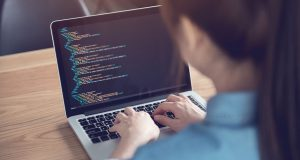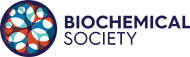# Practical python for beginnersPractical Python for beginners Summary:

An increase in the complexity and scale of biochemical data has created many opportunities for biochemists to exploit data visualisation, modelling and machine learning methods in knowledge discovery. Leveraging such power requires at least some coding which can be a daunting prospect for many biochemists. Practical Python for beginners: a Biochemist’s guide aims to teach the core skills which underlie the application of Python to complex, real-world research problems.

Following the course, the successful learner will be able to:

• Explain the rationale for scripting
• Use the IPython command line as a calculator and to assign variables
• Use the basic data types and some simple functions
• Create lists and select elements from them
• Explain what a library is, import a Python library and use functions it contains
• Read biochemical data from a file into a Python
• Understand computing concepts such as what is meant by the working directory, absolute and relative paths and be able to apply these concepts to data import

Analyse and visualise biochemical data using powerful Python packages such as NumPy, Pandas, Sklearn and Matplotlib

Nov 07 2022

### Time

All of the day